# 可分离卷积

## Spatial Separable Convolutions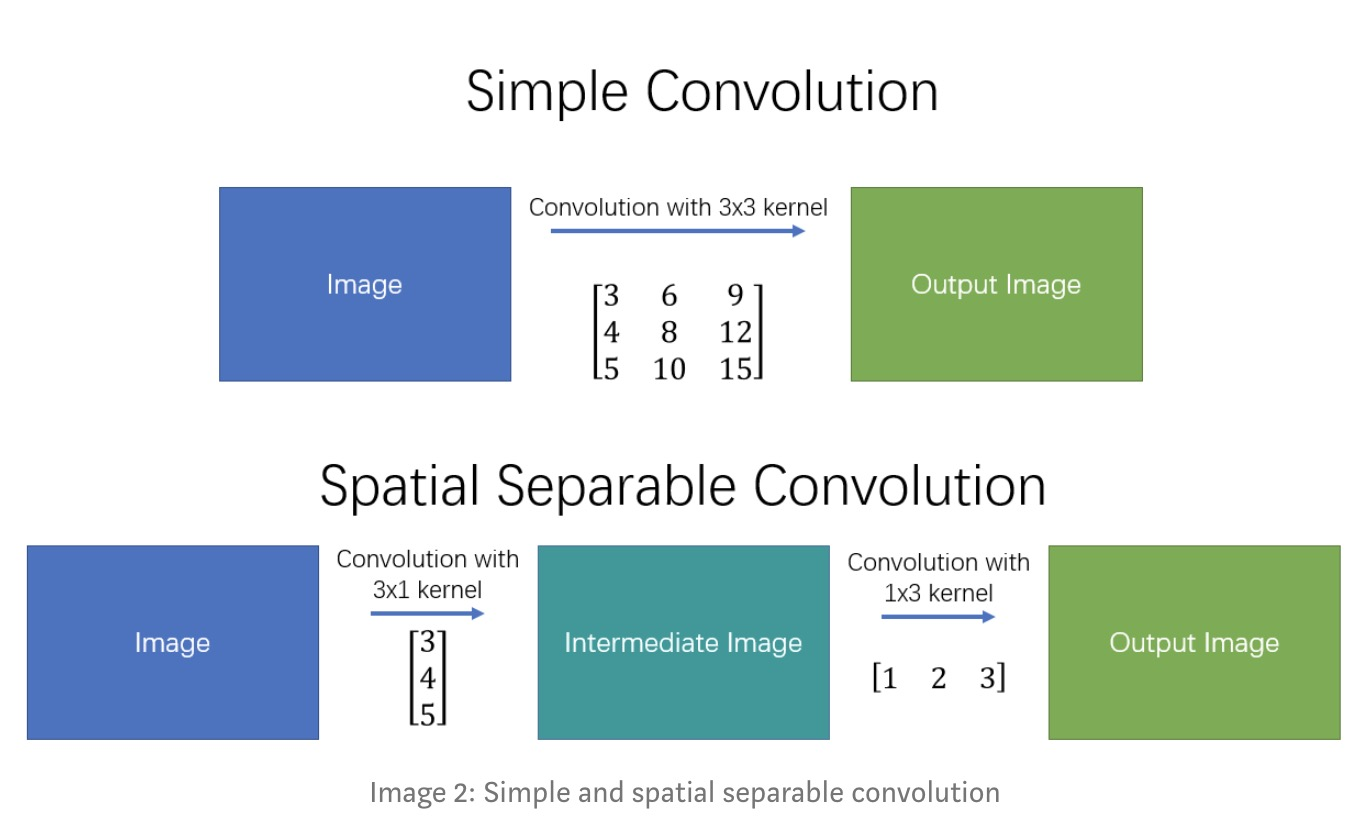## Depthwise Separable Convolutions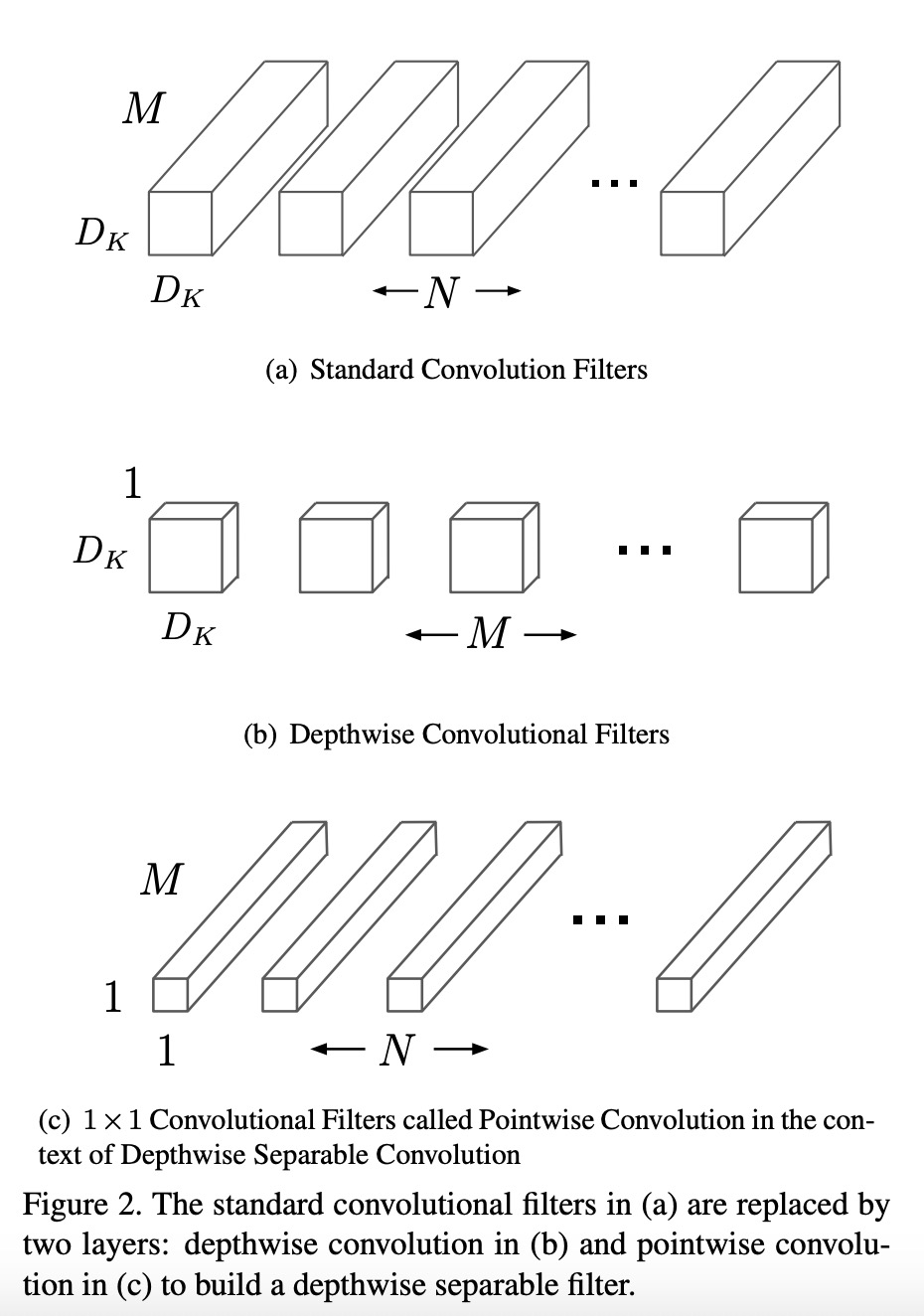• 图a)中为标准卷积，单个卷积核大小$D_K * D_K * M$, 输入通道数为$M$,输出通道数$N$.
• 图b)中将上述标准卷积分离为深度卷积，单个卷积核$D_K * D_K * 1$，共$M$个，以及$N$个$1 * 1 * M$的点卷积.

### Normal Convolution: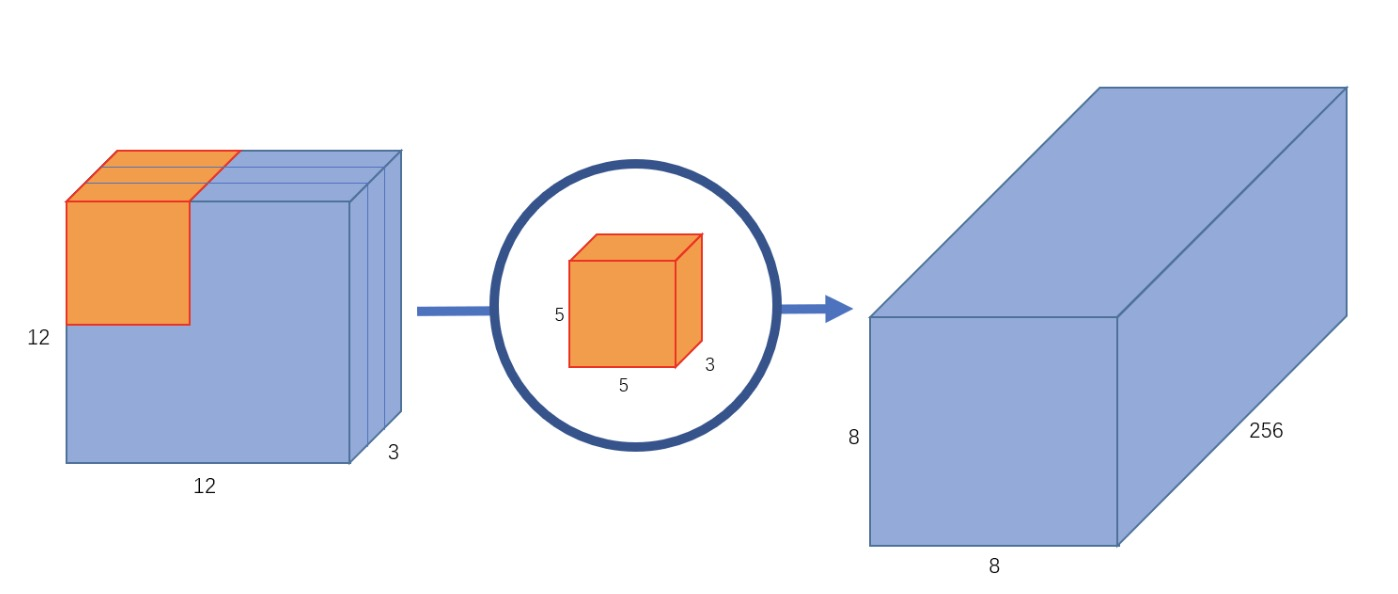### Depthwise Separable Convolutions

#### Part 1 — Depthwise Convolution: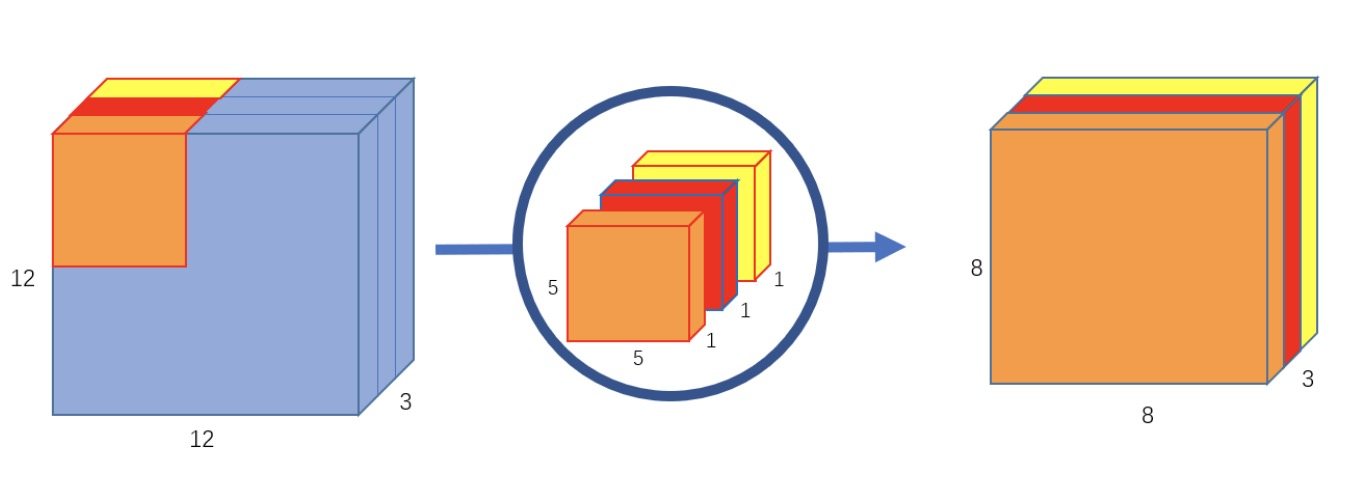### Part 2 — Pointwise Convolution: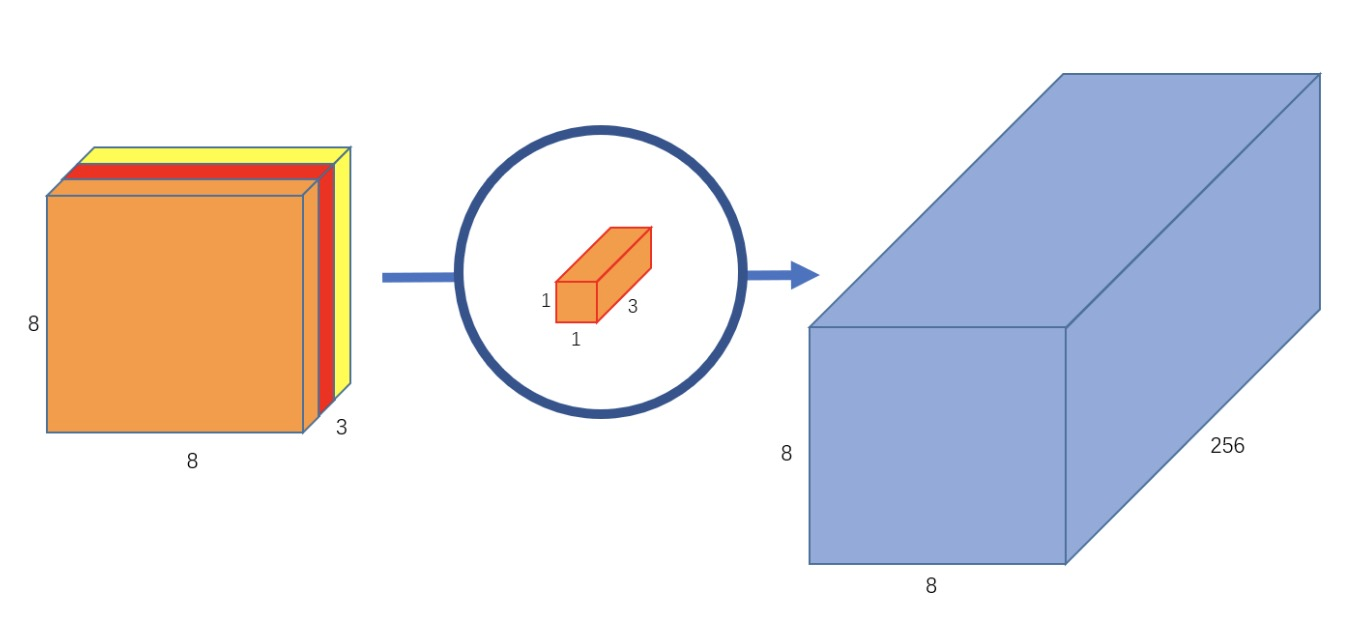### analysis

the main difference is this: in the normal convolution, we are transforming the image 256 times. And every transformation uses up 5x5x3x8x8=4800 multiplications. In the separable convolution, we only really transform the image once — in the depthwise convolution. Then, we take the transformed image and simply elongate it to 256 channels. Without having to transform the image over and over again, we can save up on computational power. ref

### usage

• SeparableConv2D： 即为完整的深度可分离卷积
• DepthwiseConv2D： 深度可分离卷积的第一部分深度卷积

ref

MobileNets